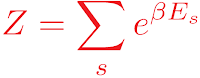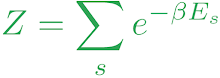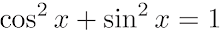## LaTuXiT

If you've ever given a scientific presentation using macOS, chances are you've run across a very handy program called LaTeXiT. This utility allows you to create nicely cropped LaTeX typset equations that can be dragged and dropped into slides. A nice feature is the use of an equation library, allowing the user to keep track of their LaTeXiT history, make modifications to existing equations, and share equations between computers. Even neater is the LinkBack feature that allows image files that have been generated with LaTeXiT to be re-opened for editing when they are dragged back into the application. That way you can drag an equation from a Keynote slide into LaTeXiT, make some edits, then drag the new image back into the slide. This is really handy when you make mistakes, or when dealing with lots of equations with slight variations.

There isn't anything quite so slick for Linux, at least anything that functions as a stand-alone package. There is KFormula, but this is now part of the Calligra suite (formerly KOffice). Since I don't use KDE or Calligra, and don't want to pull a bunch of unwanted dependencies, I decided to write something a little more lightweight.

My solution, LaTuXiT, a simple Bash script that requires no dependencies outside of the standard Tex Live core (other than Ghostscript for additional PostScript support). The script replicates two of LaTeXiT's key features: an equation library, and a reverse lookup feature to allow editing of existing equations. LaTuXiT works by hashing each equation string and building a library. Metadata written as comments in the output PDF and PostScript images allows LaTuXiT to match an image to an equation in the library. LaTuXiT has full RGB color support and also accepts any of the 68 standard colors known to dvips. Equations can be processed using several of the standard amsmath equation environments, and using custom LaTeX pre- and post-amble files. Due to its command-line nature, LaTuXiT provides a handy way of processing many equations from a batch script. For more details on the workings of LaTuXiT, please visit its GitHub page.

## Basic usage

Suppose we want a nice red image of the canonical partition function... here's a short example of a possible LaTuXiT workflow.

latuxit -e 'Z=\sum_s e^{\beta E_s}' -c 'Red'

There should now be two files in the working directory: latuxit.pdf, and latuxuit.ps. Let's open latuxit.pdf and see what it looks like.Whoops, it looks like we missed a minus sign, and how about we make the equation green instead. The following command will extract equation metadata from latuxit.pdf and open the equation in an editor where it can be corrected. Easy!

latuxit latuxit.pdf -c '{102,255,0}'

Let's check the modified image. Snazzy.## Searching the equation library

Suppose you want to edit an equation but you've lost the image file. You can kind of remember the syntax, but it's long and complicated so you don't really fancy attempting to type it out again. What do you do then?

Thankfully LaTuXiT offers a solution. As a starting point you could simply run LaTuXiT in "search" mode

latuxit -s 'sin'

The output shows a list of matching hashes from the library and the corresponding equations. Say the first match is the equation that we want. To reprocess it we can simply run LaTuXiT in hash mode. The hash string that is passed as a command-line argument should be long enough to ensure a unique match. LaTuXiT will abort if multiple matches are found. The equation will be opened in an editor so that it can be modified prior to processing.